Balbharti Maharashtra Board Class 5 Maths Solutions Chapter 5 Fractions Problem Set 22 Textbook Exercise Important Questions and Answers.

## Maharashtra State Board Class 5 Maths Solutions Chapter 5 Fractions Problem Set 22

Question 1.

$$\text { (1) } \frac{1}{8}+\frac{3}{4}$$
Solution:
The smallest common multiple of 4 and 8 is 8. So making 8 is the common denominator of the given fractions.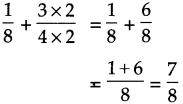$$\frac{7}{8}$$$$\text { (2) } \frac{2}{21}+\frac{3}{7}$$
Solution:
21 is the multiple of 7. So making 21 as denominator of both the fractions.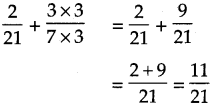$$\frac{11}{21}$$

$$\text { (3) } \frac{2}{5}+\frac{1}{3}$$
Solution:
Least common multiple of 5 and 3 is 15. So making common denominator of both the fractions 15.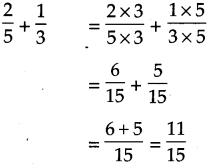$$\frac{11}{15}$$

$$\text { (4) } \frac{2}{7}+\frac{1}{2}$$
Solution:
Smallest common multiple of 2 and 7 is 14. So, making denominator of both the fractions 14.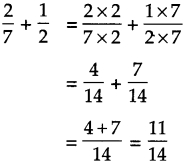$$\frac{11}{14}$$$$\text { (5) } \frac{3}{9}+\frac{3}{5}$$
Solution:
Smallest common multiple of 9 and 5 is 45.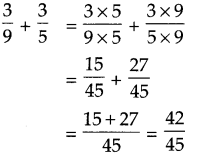$$\frac{42}{45}$$

Question 2.
Subtract the following:

$$\text { (1) } \frac{3}{10}-\frac{1}{20}$$
Solution:
20 is the multiples of 10. So,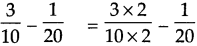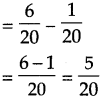$$\frac{5}{20}$$

$$\text { (2) } \frac{3}{4}-\frac{1}{2}$$
Solution:
4 is the multiple of 2. So,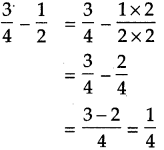$$\frac{1}{4}$$$$\text { (3) } \frac{6}{14}-\frac{2}{7}$$
Solution:
14 is the multiples of 7. So,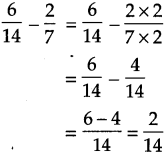$$\frac{2}{14}$$

$$\text { (4) } \frac{4}{6}-\frac{3}{5}$$
Solution:
Smallest common multiple of 6 and 5 is 30. So,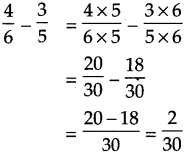$$\frac{2}{30}$$

$$\text { (5) } \frac{2}{7}-\frac{1}{4}$$
Solution:
Smallest common multiple of 7 and 4 is 28.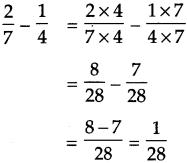$$\frac{1}{28}$$A fraction of a collection and a multiple of a fraction

$$\frac{1}{4}$$ of a collection of 20 dots – $$\frac{1}{2}$$ of a collection of 20 dots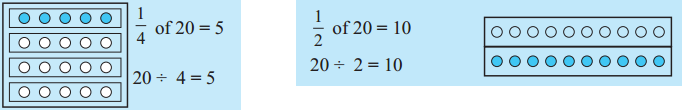$$\frac{3}{4}$$ of a collection of 20 dots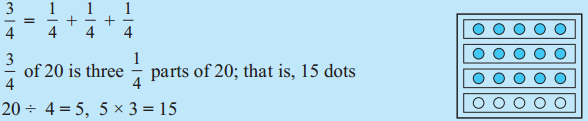Twice 5 is 10 – $$\frac{1}{2}$$ times 10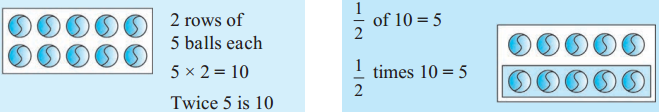Thrice 5 – $$\frac{1}{3}$$ times 15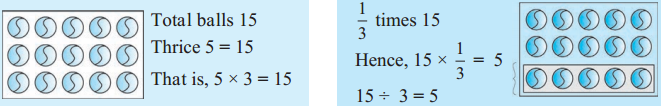$$\frac{1}{3}$$ times 15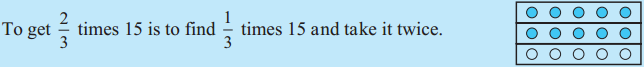Meena has 5 rupees. Tina has twice as many rupees. That is, Tina has 5 × 2 = 10 rupees. Meena has half as many rupees as Tina, that is, $$\frac{1}{2}$$ of 10, or, 5 rupees.

Ramu has to travel a distance of 20 km. If he has travelled $$\frac{4}{5}$$ of the distance by car, how many kilometres did he travel by car?
$$\frac{4}{5}$$ of 20 km is 20 × $$\frac{4}{5}$$. So, we take $$\frac{1}{5}$$ of 20, 4 times.
$$\frac{1}{5}$$ of 20 = 4. 4 times 4 is 4 × 4 = 16.
It means that 20 × $$\frac{4}{5}$$ = 16.
Ramu travelled a distance of 16 kilometres by car.

$$\text { (1) } \frac{5}{6}+\frac{1}{12}$$
Solution:
12 is the multiple of 6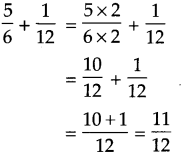$$\frac{11}{12}$$$$\text { (2) } \frac{1}{9}+\frac{2}{3}$$
Solution:
Here 9 is the multiples of 3. So, making like fractions of denominator 9, we get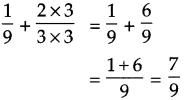$$\frac{7}{9}$$

Subtract the following:

$$\text { (1) } \frac{4}{9}-\frac{2}{5}$$
Solution:
Common multiple of 9 and 5 is 45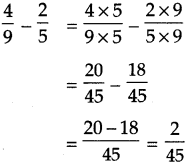$$\frac{2}{45}$$$$\text { (2) } \frac{1}{2}+\frac{3}{4}-\frac{7}{8}$$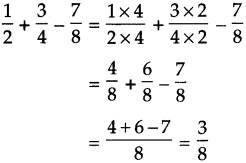$$\frac{3}{8}$$